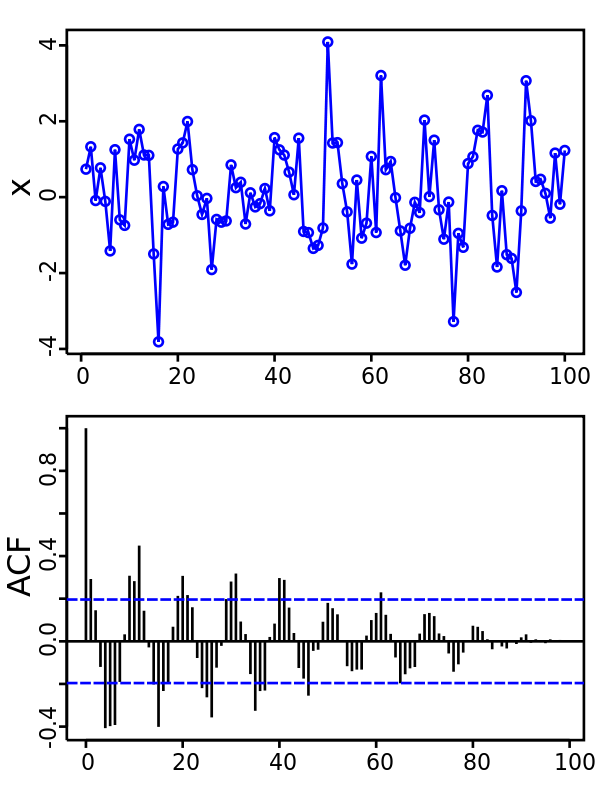Related Tags

python
auto
correlation
plot

# What is autocorrelation in Python?Educative Answers Team

Autocorrelation measures a set of current values against a set of past values to see if they correlate. It is heavily used in time series analysis and forecasting.

We can calculate the correlation for current time-series​ observations with observations of previous time steps called lags.

A plot of the autocorrelation of a time series is called the Autocorrelation Function (ACF).An example autocorrelation plot. Sharp peaks indicate a sharp correlation in time series, whereas shorter peaks indicate little correlation in the time series.

Autocorrelation plots graphically summarize the relationship between an observation in a time series and an observation at a prior time.

## Plot Autocorrelation of data in Python

The code to graphically visualize the Autocorrelation of data is given below. The plot_acf function takes in two inputs: the data column and the value for lag.

from statsmodels.graphics import tsaplots

# Display the autocorrelation plot of your time series
fig = tsaplots.plot_acf(co2_levels['co2'], lags=24)
plt.show()

RELATED TAGS

python
auto
correlation
plot